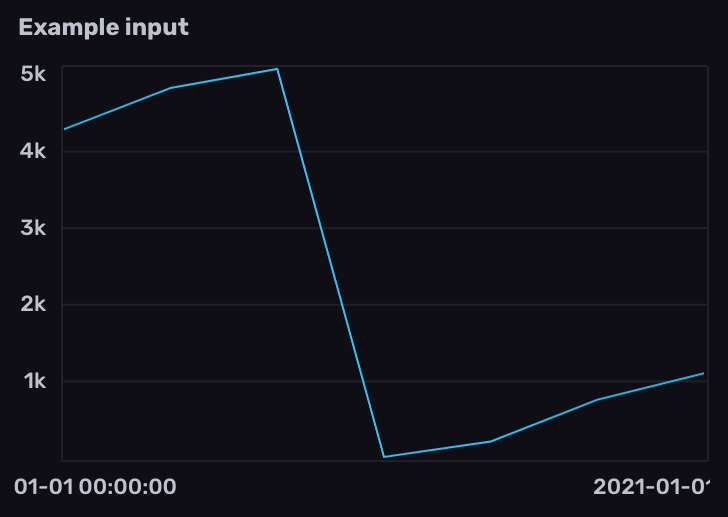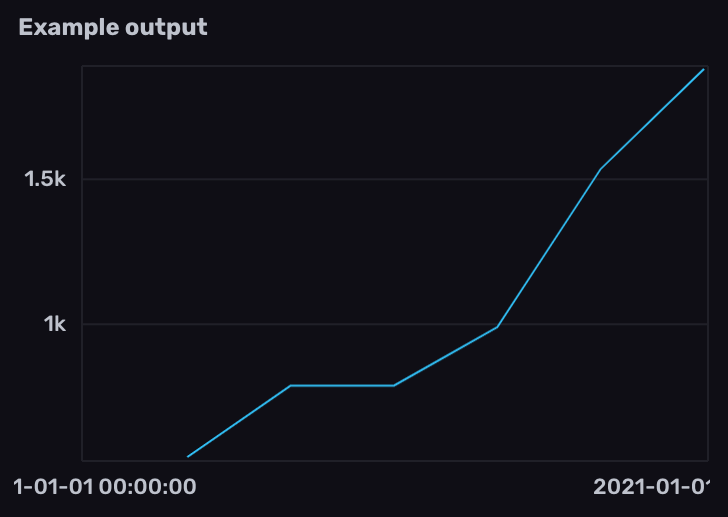# Work with Prometheus counters

Use Flux to query and transform Prometheus counter metrics stored in InfluxDB.

A counter is a cumulative metric that represents a single monotonically increasing counter whose value can only increase or be reset to zero on restart.

Prometheus metric types

##### Example counter metric in Prometheus format
``````# HELP example_counter_total Total representing an example counter metric
# TYPE example_counter_total counter
example_counter_total 282327
``````

Because counters can periodically reset to 0, any query involving counter metrics should normalize the data to account for counter resets before further processing.

The examples below include example data collected from the InfluxDB OSS 2.x `/metrics` endpoint using `prometheus.scrape()` and stored in InfluxDB.

#### Prometheus metric parsing formats

Query structure depends on the Prometheus metric parsing format used to scrape the Prometheus metrics. Select the appropriate metric format version below.

## Normalize counter resets

1. Filter results by the `prometheus` measurement and counter metric name field.
2. Use `increase()` to normalize counter resets. `increase()` returns the cumulative sum of positive changes in column values.

`increase()` accounts for counter resets, but may lose some precision on reset depending on your scrape interval. On counter reset, `increase()` assumes no increase.

``````from(bucket: "example-bucket")
|> range(start: -1m)
|> filter(fn: (r) => r._measurement == "prometheus" and r._field == "http_query_request_bytes")
|> increase()
``````View example input and output data

1. Filter results by the counter metric name measurement and `counter` field.
2. Use `increase()` to normalize counter resets. `increase()` returns the cumulative sum of positive changes in column values.

`increase()` accounts for counter resets, but may lose some precision on reset depending on your scrape interval. On counter reset, `increase()` assumes no increase.

``````from(bucket: "example-bucket")
|> range(start: -1m)
|> filter(fn: (r) => r._measurement == "http_query_request_bytes" and r._field == "counter")
|> increase()
``````View example input and output data

## Calculate changes between normalized counter values

Use `difference()` with normalized counter data to return the difference between subsequent values.

``````from(bucket: "example-bucket")
|> range(start: -1m)
|> filter(fn: (r) => r._measurement == "prometheus" and r._field == "http_query_request_bytes")
|> increase()
|> difference()
``````View example input and output data

``````from(bucket: "example-bucket")
|> range(start: -1m)
|> filter(fn: (r) => r._measurement == "http_query_request_bytes" and r._field == "counter")
|> increase()
|> difference()
``````View example input and output data

## Calculate the rate of change in normalized counter values

Use `derivative()` to calculate the rate of change between normalized counter values. By default, `derivative()` returns the rate of change per second. Use the `unit` parameter to customize the rate unit.

``````from(bucket: "example-bucket")
|> range(start: -1m)
|> filter(fn: (r) => r._measurement == "prometheus" and r._field == "http_query_request_bytes")
|> increase()
|> derivative()
``````View example input and output data

``````from(bucket: "example-bucket")
|> range(start: -1m)
|> filter(fn: (r) => r._measurement == "http_query_request_bytes" and r._field == "counter")
|> increase()
|> derivative()
``````View example input and output data

## Calculate the average rate of change in specified time windows

To calculate the average rate of change in normalized counter values in specified time windows:

1. Import the `experimental/aggregate` package.

2. Use `aggregate.rate()` to calculate the average rate of change per time window.

``````import "experimental/aggregate"

from(bucket: "example-bucket")
|> range(start: -1m)
|> filter(fn: (r) => r._measurement == "prometheus" and r._field == "http_query_request_bytes")
|> increase()
|> aggregate.rate(every: 15s, unit: 1s)
``````View example input and output data

``````import "experimental/aggregate"

from(bucket: "example-bucket")
|> range(start: -1m)
|> filter(fn: (r) => r._measurement == "http_query_request_bytes" and r._field == "counter")
|> increase()
|> aggregate.rate(every: 15s, unit: 1s)
``````View example input and output data

### Introducing InfluxDB Clustered

A highly available InfluxDB 3.0 cluster on your own infrastructure.

InfluxDB Clustered is a highly available InfluxDB 3.0 cluster built for high write and query workloads on your own infrastructure.

InfluxDB Clustered is currently in limited availability and is only available to a limited group of InfluxData customers. If interested in being part of the limited access group, please contact the InfluxData Sales team.

### The future of Flux

Flux is going into maintenance mode. You can continue using it as you currently are without any changes to your code.

Flux is going into maintenance mode and will not be supported in InfluxDB 3.0. This was a decision based on the broad demand for SQL and the continued growth and adoption of InfluxQL. We are continuing to support Flux for users in 1.x and 2.x so you can continue using it with no changes to your code. If you are interested in transitioning to InfluxDB 3.0 and want to future-proof your code, we suggest using InfluxQL.

For information about the future of Flux, see the following:

### State of the InfluxDB Cloud Serverless documentation

InfluxDB Cloud Serverless documentation is a work in progress.

The new documentation for InfluxDB Cloud Serverless is a work in progress. We are adding new information and content almost daily. Thank you for your patience!

If there is specific information you’re looking for, please submit a documentation issue.